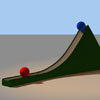#### You may also like### Whoosh

A ball whooshes down a slide and hits another ball which flies off the slide horizontally as a projectile. How far does it go?

# Dam Busters 2

##### Age 16 to 18 Challenge Level:

This is a computationally involved problem, but draws together many strands of mechanics. A full solution is given here.

The correct equation is $$h =-\frac{1}{2}g\left[\frac{D}{V}-\sqrt{\frac{2H}{g}}\right]^2 + eg\sqrt{\frac{2H}{g}}\left[\frac{D}{V}-\sqrt{\frac{2H}{g}}\right]< T\;.$$ The parts in the brackets and the power of $g$ are obtained by dimensional analysis (the coefficient of restitution $e$, being a ratio of velocities, is dimensionless). The overall signs are obtained by noting that the first part of the motion is down and the second up.

Doug writes:

For the first part of the problem, use 'dimensional analysis'. Start by looking at the dimensions of the equation. Summed terms must have the same dimensions. There is only one term involving a single unknown quantity, so look at this: we must have
$$[h] = [eg\sqrt{X}\times{\sqrt{X}}]\;.$$

This shows that $X$ must have dimensions of time squared. Thus $X = \frac{2H}{g}$.

We now know that the first term inside the brackets must be $\frac{D}{V}$, to have the same units as $\sqrt{X}$. And we can see that the power of the first $g$ must be +1, so that the second main term has units of distance.

Finally we need to find whether the operand between the main terms is plus or minus. The first main term is an overall subtraction, so the second term must be an overall addition, for $h$ to be positive. So I tackled this by considering whether the expression inside the brackets is positive or negative. Taking the values for $V$, $D$ and $H$ given in Dam Busters 1, the expression inside the brackets evaluates to just negative, -1.9. But we have been told that this bouncing method allows release at a greater distance, which will cause this term to be increased. Thus the expression inside the brackets will be positive, and so also the operand between the main terms. It also makes physical sense that the term that contains $e$, the coefficient of restitution (bouncing), would be an addition.

Cul-de-sac:

For part (b) "explore $H$ & $D$", I started by looking at the formula as a quadratic in $\frac{D}{V} - \sqrt{X} = Y$ which resolves to
$$Y^2 - 2e\sqrt{X}Y + 2\frac{h}{g} = 0 \Rightarrow Y = e\sqrt{X} \pm \sqrt{e^2 X - 2\frac{h}{G}}\;.$$

For real roots, this gives $h < \frac{e^2H}{4}$, but for the values given, this gives $h < 32 \mathrm{\ m}$, which is below the base. I believe this is invalid since $\sqrt{X}$ is part of the subject and also a coefficient. I had concerns about that when I started down the cul-de-sac, but explored it anyway.

I then decided to expand the equation to eliminate the multiple $\sqrt{X}$, and got to
$$\frac{1}{2} g \left( \sqrt{X} (1+2e) - \frac{D}{V} \right) \left( \frac{D}{V} - \sqrt{X} \right)\;.$$

With hindsight, a new quadratic in $\frac{D}{V}$ does make sense here, and gives
$$\frac{D}{V} = \frac{2\sqrt{X}(1+e) \pm \sqrt{4X(1+e)^2 - 4 \left[ X(1+2e) + 2\frac{h}{g} \right]}}{2}\;.$$

For real roots, $(1+e)^2 > (1+2e) + 2\frac{h}{X}g$, so $e > \sqrt{\frac{2h}{Xg}}$, and so for minimum $h$ $\left(100\textrm{\ m}\right)$, and the values given, $e > \frac{1}{\sqrt{2}} \approx 0.707\;.$

Having learned some more about the problem, I got back to the 2nd part "explore $H$ & $D$".

There are 4 "unknowns": $H$, $D$, $V$, and $h$, but you are given maximum values for $H$ and $V$, so the first thought is to find $D$ with these values, and a sensible value of $h$, especially given the sense that the plane would want to maintain maximum speed, and wouldn't want to fly much lower than the maximum, for safety reasons. $V = 800 \mathrm{\ km/h}$, and we "multiply by one" to change the units (1000 m / 1 km is one, and 1 hr / 3600 s is one) to get $\frac{2000}{9}\mathrm{\ ms^{-1}}$. Solving for $\frac{D}{V}$, we find $D = 3087\mathrm{\ m}$ or $2024\mathrm{\ m}$ when $h=100\mathrm{\ m}$, and $D = 2556\mathrm{\ m}$ when $h = 128 \mathrm{\ m}$.

$\underline{Derivation}$

The equation is derived as follows:

Suppose the bomb is dropped from a height $H$ with horizontal speed $V$. It's equation of motion in the vertical direction is $x(t) = H - \frac{1}{2}gt^2$. The bomb bounces when $x(t) = 0$ i.e. when $t = \sqrt{\frac{2H}{g}}$. We set $\frac{2H}{g} = X$. The bomb has vertical speed $-g\sqrt{X}$ just before the bounce, so has vertical speed $eg\sqrt{X}$ just after the bounce.

The bomb has travelled a distance $V\sqrt{X}$ when it bounces. If we reset $t = 0$ on the bounce, then the equation of motion of the bomb is $x(t) = egt\sqrt{X} - \frac{1}{2}gt^2$.

At a distance $D > V\sqrt{X}$ the bomb has been travelling for a time $t = \frac{D - V\sqrt{X}}{V} = \frac{D}{V} - \sqrt{X}$ since the bounce. It is therefore at a height $$h = x\Big(\frac{D}{V} - \sqrt{X}\Big) = -\frac{1}{2}g\Big[\frac{D}{V} - \sqrt{X}\Big]^2 + eg\sqrt{X}\Big[\frac{D}{V} - \sqrt{X}\Big]\;.$$.

Observe that we can write
$$\begin{eqnarray} h &=&-\frac{1}{2}g\left[\frac{D}{V} - \sqrt{X} - 2e\sqrt{X}\right]\left(\frac{D}{V} - \sqrt{X}\right)\\ &=&-\frac{1}{2}g\left(\frac{D}{V} - (1 + 2e)\sqrt{X}\right)\left(\frac{D}{V} - \sqrt{X}\right)\;. \end{eqnarray}$$
If we want to investigate the heights and distances for which we will get a hit, it might help to note that $h$ is given by a quadratic equation in $\frac{D}{V}$ with roots at $\sqrt{X}$ and $(1 + 2e)\sqrt{X}\ldots$